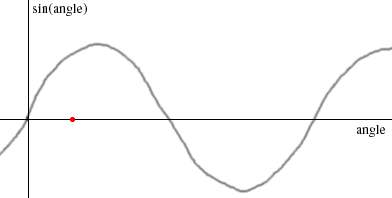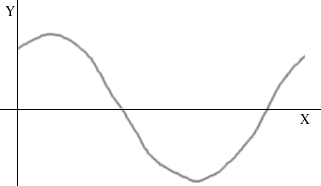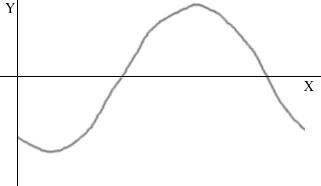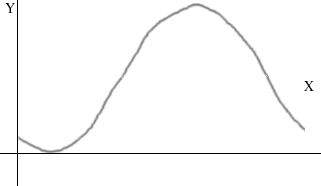Quandaries and Queries Name: Eman Who is asking: Student Level: Secondary Hi there, I am stuck on this question having many parts, the problem is I know how to do all the parts once i get through the fist line!!! here it is..(the first part) Q : Sketch the graph of y = 1 - sin(x+60). for 0 <= x<= 360, giving the coordinates of the maximum and minimum points and the pints where the curves crosses the y axis. <= means 'less than equal to' This is an AS level question. Please help me soon, because I am studying through correspondense and there are not many to help me here. Thank you so much!!! Hi Eman, I am going to build the graph from the graph of the sine function. I see the sine function as the graphy = sin(angle) where the graph crosses the horizontal axis when the angle is 0, 180, 360, ... (I put a red dot on the horizontal axis at 60 degrees.) For a graph of sin(x + 60) with 0 <= x<= 360, the angle starts at 60 degrees and ends at 360 + 60 = 420 degrees. Thus the graph of y = sin(x + 60) for 0 <= x<= 360 isy = sin(x + 60) But you need y = - sin(x + 60), so all the positive values of y become negative and the negative values become positive. Hence the graph isy = - sin(x + 60) One last step. What you want is y = 1 - sin(x + 60), so you need to add 1 to each y-value on the graph. Hence the final graph isy = 1 - sin(x + 60) Cheers, Penny Go to Math Central